# The Unit Commitment Problem (UCP)¶

This tutorial includes everything you need to set up IBM Decision Optimization CPLEX Modeling for Python (DOcplex), build a Mathematical Programming model, and get its solution by solving the model on the cloud with IBM ILOG CPLEX Optimizer.

When you finish this tutorial, you’ll have a foundational knowledge of Prescriptive Analytics.

This notebook is part of Prescriptive Analytics for Python

It requires either an installation of CPLEX Optimizers or it can be run on IBM Watson Studio Cloud (Sign up for a free IBM Cloud account and you can start using Watson Studio Cloud right away).

• The Model estimates the lower cost of generating electricity within a given plan. Depending on the demand for electricity, we turn on or off units that generate power and which have operational properties and costs.
• The Unit Commitment Problem answers the question “Which power generators should I run at which times and at what level in order to satisfy the demand for electricity?”. This model helps users to find not only a feasible answer to the question, but one that also optimizes its solution to meet as many of the electricity company’s overall goals as possible.

## How decision optimization can help¶

• Prescriptive analytics (decision optimization) technology recommends actions that are based on desired outcomes. It takes into account specific scenarios, resources, and knowledge of past and current events. With this insight, your organization can make better decisions and have greater control of business outcomes.

• Prescriptive analytics is the next step on the path to insight-based actions. It creates value through synergy with predictive analytics, which analyzes data to predict future outcomes.

• Prescriptive analytics takes that insight to the next level by suggesting the optimal way to handle that future situation. Organizations that can act fast in dynamic conditions and make superior decisions in uncertain environments gain a strong competitive advantage.

With prescriptive analytics, you can:

• Automate the complex decisions and trade-offs to better manage your limited resources.
• Take advantage of a future opportunity or mitigate a future risk.
• Proactively update recommendations based on changing events.
• Meet operational goals, increase customer loyalty, prevent threats and fraud, and optimize business processes.

## Checking minimum requirements¶

This notebook uses some features of pandas that are available in version 0.17.1 or above.

## Use decision optimization¶

### Step 1: Import the library¶

Run the following code to the import the Decision Optimization CPLEX Modeling library. The DOcplex library contains the two modeling packages, Mathematical Programming (docplex.mp) and Constraint Programming (docplex.cp).

### Step 2: Model the data¶

#### Load data from a pandas DataFrame¶

Data for the Unit Commitment Problem is provided as a pandas DataFrame. For a standalone notebook, we provide the raw data as Python collections, but real data could be loaded from an Excel sheet, also using pandas.

Update the configuration of notebook so that display matches browser window width.

#### Available energy technologies¶

The following df_energy DataFrame stores CO2 cost information, indexed by energy type.

co2_cost
coal 30
gas 5
diesel 15
wind 0

The following df_units DataFrame stores common elements for units of a given technology.

energy initial min_gen max_gen operating_max_gen min_uptime min_downtime ramp_up ramp_down start_cost fixed_cost variable_cost
coal1 coal 400 100.00 425 400 15 9 212.0 183.0 5000 208.610 22.536
coal2 coal 350 140.00 365 350 15 8 150.0 198.0 4550 117.370 31.985
gas1 gas 205 78.00 220 205 6 7 101.2 95.6 1320 174.120 70.500
gas2 gas 52 52.00 210 197 5 4 94.8 101.7 1291 172.750 69.000
gas3 gas 155 54.25 165 155 5 3 58.0 77.5 1280 95.353 32.146
gas4 gas 150 39.00 158 150 4 2 50.0 60.0 1105 144.520 54.840
diesel1 diesel 78 17.40 90 78 3 2 40.0 24.0 560 54.417 40.222
diesel2 diesel 76 15.20 87 76 3 2 60.0 45.0 554 54.551 40.522
diesel3 diesel 0 4.00 20 20 1 1 20.0 20.0 300 79.638 116.330
diesel4 diesel 0 2.40 12 12 1 1 12.0 12.0 250 16.259 76.642

### Step 3: Prepare the data¶

The pandas merge operation is used to create a join between the df_units and df_energy DataFrames. Here, the join is performed based on the ‘energy’ column of df_units and index column of df_energy.

By default, merge performs an inner join. That is, the resulting DataFrame is based on the intersection of keys from both input DataFrames.

energy initial min_gen max_gen operating_max_gen min_uptime min_downtime ramp_up ramp_down start_cost fixed_cost variable_cost co2_cost
units
coal1 coal 400 100.00 425 400 15 9 212.0 183.0 5000 208.610 22.536 30
coal2 coal 350 140.00 365 350 15 8 150.0 198.0 4550 117.370 31.985 30
gas1 gas 205 78.00 220 205 6 7 101.2 95.6 1320 174.120 70.500 5
gas2 gas 52 52.00 210 197 5 4 94.8 101.7 1291 172.750 69.000 5
gas3 gas 155 54.25 165 155 5 3 58.0 77.5 1280 95.353 32.146 5

The demand is stored as a pandas Series indexed from 1 to the number of periods.

nb periods = 192

<matplotlib.axes._subplots.AxesSubplot at 0x23fbad3d588>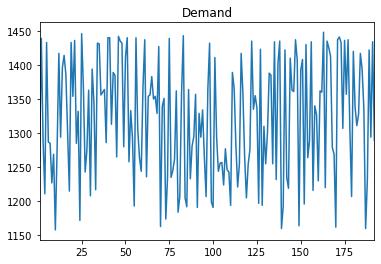### Step 4: Set up the prescriptive model¶

* system is: Windows 64bit
* Python version 3.7.3, located at: c:\local\python373\python.exe
* docplex is present, version is (2, 11, 0)
* pandas is present, version is 0.25.1


#### Create the DOcplex model¶

The model contains all the business constraints and defines the objective.

#### Define the decision variables¶

Decision variables are:

• The variable in_use[u,t] is 1 if and only if unit u is working at period t.
• The variable turn_on[u,t] is 1 if and only if unit u is in production at period t.
• The variable turn_off[u,t] is 1 if unit u is switched off at period t.
• The variable production[u,t] is a continuous variables representing the production of energy for unit u at period t.
Model: ucp
- number of variables: 7680
- binary=3840, integer=0, continuous=3840
- number of constraints: 0
- linear=0
- parameters: defaults
- problem type is: MILP

in_use turn_on turn_off production
units periods
coal1 1 in_use_coal1_1 turn_on_coal1_1 turn_off_coal1_1 p_coal1_1
2 in_use_coal1_2 turn_on_coal1_2 turn_off_coal1_2 p_coal1_2
3 in_use_coal1_3 turn_on_coal1_3 turn_off_coal1_3 p_coal1_3
4 in_use_coal1_4 turn_on_coal1_4 turn_off_coal1_4 p_coal1_4
5 in_use_coal1_5 turn_on_coal1_5 turn_off_coal1_5 p_coal1_5

##### Linking in-use status to production¶

Whenever the unit is in use, the production must be within the minimum and maximum generation.

in_use turn_on turn_off production min_gen max_gen
units periods
coal1 1 in_use_coal1_1 turn_on_coal1_1 turn_off_coal1_1 p_coal1_1 100.0 425
2 in_use_coal1_2 turn_on_coal1_2 turn_off_coal1_2 p_coal1_2 100.0 425
3 in_use_coal1_3 turn_on_coal1_3 turn_off_coal1_3 p_coal1_3 100.0 425
4 in_use_coal1_4 turn_on_coal1_4 turn_off_coal1_4 p_coal1_4 100.0 425
5 in_use_coal1_5 turn_on_coal1_5 turn_off_coal1_5 p_coal1_5 100.0 425
0.25.1

##### Initial state¶

The solution must take into account the initial state. The initial state of use of the unit is determined by its initial production level.

Model: ucp
- number of variables: 7680
- binary=3840, integer=0, continuous=3840
- number of constraints: 3860
- linear=3860
- parameters: defaults
- problem type is: MILP

##### Ramp-up / ramp-down constraint¶

Variations of the production level over time in a unit is constrained by a ramp-up / ramp-down process.

We use the pandas groupby operation to collect all decision variables for each unit in separate series. Then, we iterate over units to post constraints enforcing the ramp-up / ramp-down process by setting upper bounds on the variation of the production level for consecutive periods.

Model: ucp
- number of variables: 7680
- binary=3840, integer=0, continuous=3840
- number of constraints: 7700
- linear=7700
- parameters: defaults
- problem type is: MILP

##### Turn on / turn off¶

The following constraints determine when a unit is turned on or off.

We use the same pandas groupby operation as in the previous constraint to iterate over the sequence of decision variables for each unit.

Model: ucp
- number of variables: 7680
- binary=3840, integer=0, continuous=3840
- number of constraints: 11520
- linear=11520
- parameters: defaults
- problem type is: MILP

##### Minimum uptime and downtime¶

When a unit is turned on, it cannot be turned off before a minimum uptime. Conversely, when a unit is turned off, it cannot be turned on again before a minimum downtime.

Again, let’s use the same pandas groupby operation to implement this constraint for each unit.

##### Demand constraint¶

Total production level must be equal or higher than demand on any period.

This time, the pandas operation groupby is performed on “periods” since we have to iterate over the list of all units for each period.

#### Express the objective¶

Operating the different units incur different costs: fixed cost, variable cost, startup cost, co2 cost.

In a first step, we define the objective as a non-weighted sum of all these costs.

The following pandas join operation groups all the data to calculate the objective in a single DataFrame.

in_use turn_on turn_off production fixed_cost variable_cost start_cost co2_cost
units periods
coal1 1 in_use_coal1_1 turn_on_coal1_1 turn_off_coal1_1 p_coal1_1 208.61 22.536 5000 30
2 in_use_coal1_2 turn_on_coal1_2 turn_off_coal1_2 p_coal1_2 208.61 22.536 5000 30
3 in_use_coal1_3 turn_on_coal1_3 turn_off_coal1_3 p_coal1_3 208.61 22.536 5000 30
4 in_use_coal1_4 turn_on_coal1_4 turn_off_coal1_4 p_coal1_4 208.61 22.536 5000 30
5 in_use_coal1_5 turn_on_coal1_5 turn_off_coal1_5 p_coal1_5 208.61 22.536 5000 30

#### Solve with Decision Optimization¶

If you’re using a Community Edition of CPLEX runtimes, depending on the size of the problem, the solve stage may fail and will need a paying subscription or product installation.

Model: ucp
- number of variables: 7680
- binary=3840, integer=0, continuous=3840
- number of constraints: 15455
- linear=15455
- parameters: defaults
- problem type is: MILP

* model ucp solved with objective = 14213082.064
*  KPI: Total Fixed Cost    = 161025.131
*  KPI: Total Variable Cost = 8865900.433
*  KPI: Total Startup Cost  = 2832.000
*  KPI: Total Economic Cost = 9029757.564
*  KPI: Total CO2 Cost      = 5183324.500
*  KPI: Total #used         = 1335.000
*  KPI: Total #starts       = 3.000


### Step 5: Investigate the solution and then run an example analysis¶

Now let’s store the results in a new pandas DataFrame.

For convenience, the different figures are organized into pivot tables with periods as row index and units as columns. The pandas unstack operation does this for us.

units coal1 coal2 diesel1 diesel2 diesel3 diesel4 gas1 gas2 gas3 gas4
periods
1 425.0 215.0 90.0 87.0 0.0 0.0 109.4 52.0 165.0 115.6
2 425.0 365.0 90.0 87.0 0.0 0.0 78.0 71.0 165.0 158.0
3 425.0 312.0 90.0 87.0 0.0 0.0 0.0 52.0 165.0 158.0
4 425.0 234.0 90.0 87.0 0.0 0.0 0.0 52.0 165.0 158.0
5 425.0 365.0 90.0 87.0 0.0 0.0 0.0 143.0 165.0 158.0

From these raw DataFrame results, we can compute derived results. For example, for a given unit and period, the reserve r(u,t) is defined as the unit’s maximum generation minus the current production.

coal1 coal2 diesel1 diesel2 diesel3 diesel4 gas1 gas2 gas3 gas4
1 0.0 150.0 0.0 0.0 20.0 12.0 110.6 158.0 0.0 42.4
2 0.0 0.0 0.0 0.0 20.0 12.0 142.0 139.0 0.0 0.0
3 0.0 53.0 0.0 0.0 20.0 12.0 220.0 158.0 0.0 0.0
4 0.0 131.0 0.0 0.0 20.0 12.0 220.0 158.0 0.0 0.0
5 0.0 0.0 0.0 0.0 20.0 12.0 220.0 67.0 0.0 0.0

Let’s plot the evolution of the reserves for the “coal2” unit:

<matplotlib.axes._subplots.AxesSubplot at 0x23fd903bf60>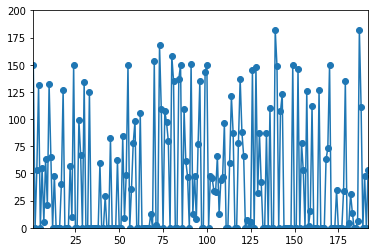Now we want to sum all unit reserves to compute the global spinning reserve. We need to sum all columns of the DataFrame to get an aggregated time series. We use the pandas sum method with axis=1 (for rows).

<matplotlib.axes._subplots.AxesSubplot at 0x23fda312710>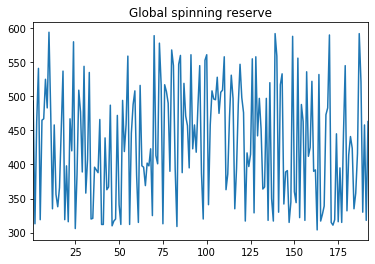#### Number of plants online by period¶

The total number of plants online at each period t is the sum of in_use variables for all units at this period. Again, we use the pandas sum with axis=1 (for rows) to sum over all units.

<matplotlib.axes._subplots.AxesSubplot at 0x23fda37f9b0>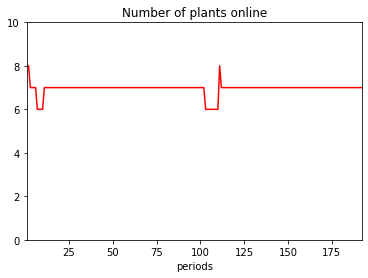#### Costs by period¶

<matplotlib.axes._subplots.AxesSubplot at 0x23fda3c7e80>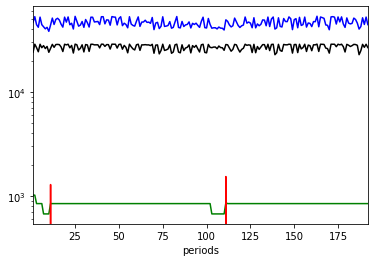#### Cost breakdown by unit and by energy¶

Text(0.5, 1.0, 'total cost by energy type')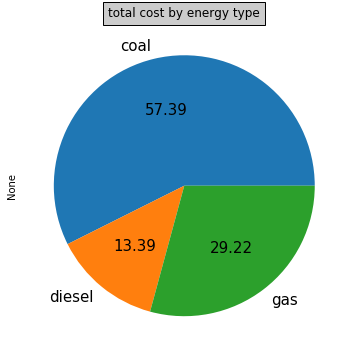### Arbitration between CO2 cost and economic cost¶

Economic cost and CO2 cost usually push in opposite directions. In the above discussion, we have minimized the raw sum of economic cost and CO2 cost, without weights. But how good could we be on CO2, regardless of economic constraints? To know this, let’s solve again with CO2 cost as the only objective.

* current CO2 cost is: 5183324.5
* current $$cost is: 9029757.56390015  * absolute minimum for CO2 cost is 3399032.0 * at this point$$$cost is 12434227.620000225  As expected, we get a significantly lower CO2 cost when minimized alone, at the price of a higher economic cost. We could do a similar analysis for economic cost to estimate the absolute minimum of the economic cost, regardless of CO2 cost. * absolute minimum for$ cost is 8887433.859000005
* at this point CO2 cost is 5375417.0


Again, the absolute minimum for economic cost is lower than the figure we obtained in the original model where we minimized the sum of economic and CO2 costs, but here we significantly increase the CO2.

But what happens in between these two extreme points?

To investigate, we will divide the interval of CO2 cost values in smaller intervals, add an upper limit on CO2, and minimize economic cost with this constraint. This will give us a Pareto optimal point with at most this CO2 value.

To avoid adding many constraints, we add only one constraint with an extra variable, and we change only the upper bound of this CO2 limit variable between successive solves.

Then we iterate (with a fixed number of iterations) and collect the cost values.

iteration #0 co2_ub=3399032.0
iteration #1 co2_ub=3438559.7
iteration #2 co2_ub=3478087.4
iteration #3 co2_ub=3517615.1
iteration #4 co2_ub=3557142.8
iteration #5 co2_ub=3596670.5
iteration #6 co2_ub=3636198.2
iteration #7 co2_ub=3675725.9
iteration #8 co2_ub=3715253.6
iteration #9 co2_ub=3754781.3
iteration #10 co2_ub=3794309.0
iteration #11 co2_ub=3833836.7
iteration #12 co2_ub=3873364.4
iteration #13 co2_ub=3912892.1
iteration #14 co2_ub=3952419.8
iteration #15 co2_ub=3991947.5
iteration #16 co2_ub=4031475.2
iteration #17 co2_ub=4071002.9
iteration #18 co2_ub=4110530.6
iteration #19 co2_ub=4150058.3
iteration #20 co2_ub=4189586.0
iteration #21 co2_ub=4229113.7
iteration #22 co2_ub=4268641.4
iteration #23 co2_ub=4308169.1
iteration #24 co2_ub=4347696.8
iteration #25 co2_ub=4387224.5
iteration #26 co2_ub=4426752.2
iteration #27 co2_ub=4466279.9
iteration #28 co2_ub=4505807.6
iteration #29 co2_ub=4545335.3
iteration #30 co2_ub=4584863.0
iteration #31 co2_ub=4624390.7
iteration #32 co2_ub=4663918.4
iteration #33 co2_ub=4703446.1
iteration #34 co2_ub=4742973.8
iteration #35 co2_ub=4782501.5
iteration #36 co2_ub=4822029.2
iteration #37 co2_ub=4861556.9
iteration #38 co2_ub=4901084.6
iteration #39 co2_ub=4940612.3
iteration #40 co2_ub=4980140.0
iteration #41 co2_ub=5019667.7
iteration #42 co2_ub=5059195.4
iteration #43 co2_ub=5098723.1
iteration #44 co2_ub=5138250.8
iteration #45 co2_ub=5177778.5
iteration #46 co2_ub=5217306.2
iteration #47 co2_ub=5256833.9
iteration #48 co2_ub=5296361.6
iteration #49 co2_ub=5335889.3
iteration #50 co2_ub=5375417.0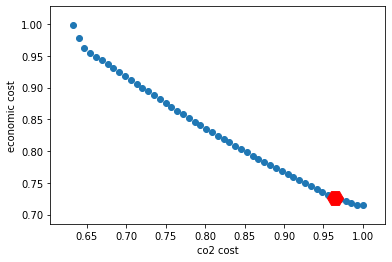This figure demonstrates that the result obtained in the initial model clearly favored economic cost over CO2 cost: CO2 cost is well above 95% of its maximum value.

## Summary¶

You learned how to set up and use IBM Decision Optimization CPLEX Modeling for Python to formulate a Mathematical Programming model and solve it with IBM Decision Optimization on Cloud.